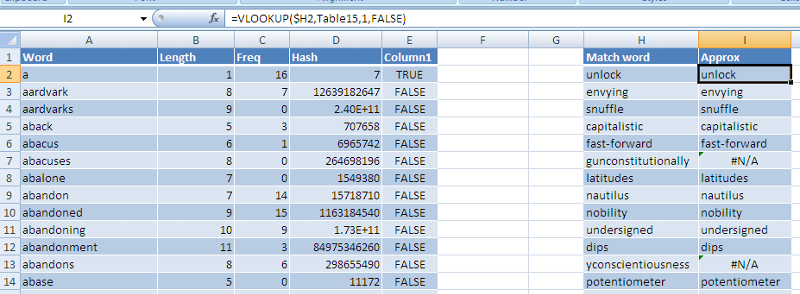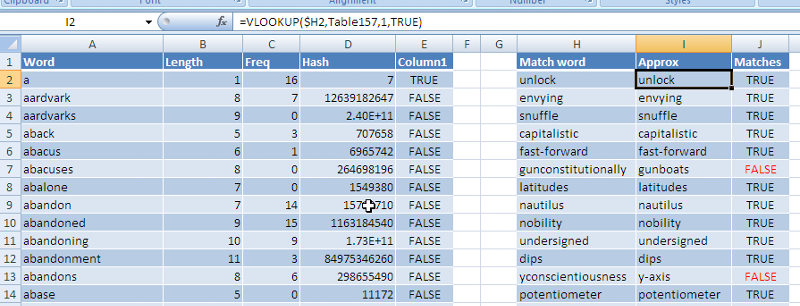# Excel performance - approximate matching with MATCH and VLOOKUP

Published on
14,455 Points
4,155 Views
8 Endorsements
Awarded
Approximate matching with VLOOKUP and MATCH seems to me to be a greatly under-used technique, and one which is vital for getting good performance out of large lookups. Until recently I would always have advised using an exact match for simplicity and, well, exactness. But after looking in more detail and doing some timings I'm now a convert to approximate matching.

http://www.experts-exchange.com/A_2637.html
http://www.experts-exchange.com/A_5511.html

For VLOOKUP it's the fourth parameter that determines the type of match. This is what Microsoft says about it:
VLOOKUP(lookup_value,table_array,col_index_num,range_lookup)

Range_lookup - A logical value that specifies whether you want VLOOKUP to find an exact match or an approximate match:
•      If TRUE or omitted, an exact or approximate match is returned. If an exact match is not found, the next largest value that is less than lookup_value is returned.
•      The values in the first column of table_array must be placed in ascending sort order; otherwise, VLOOKUP may not give the correct value.
•      If FALSE, VLOOKUP will only find an exact match. In this case, the values in the first column of table_array do not need to be sorted. If there are two or more values in the first column of table_array that match the lookup_value, the first value found is used. If an exact match is not found, the error value #N/A is returned.

For MATCH, it's the third parameter, which is similar except that it can be 0 for exact, 1 for data sorted in ascending (smallest to largest) order, or -1 for data in descending order.

What is important here is that the lookup data must be sorted to use an approximate match; this is not usually a problem as most lookups are on dates or other lists that can easily be sorted.

'Approximate matching', which is Microsoft's terminology, is a bit misleading here because it suggests that it will find just roughly the right data, but of course it's more controlled than that. It will return the correct data if it matches exactly, but if not, it returns the item that would have immediately preceded the correct data in the list if it were there (that what 'the next largest value that is less than lookup_value' means). The only time it will return #N/A is if the value you are looking for is smaller than the first value in the lookup list.

Let's look at an example that will give you some idea of the performance benefits.  It's not a typical Bank example, but I wanted to make it obvious what's going on, so the spreadsheet attached here is looking up words from a dictionary list to check whether they exist there. The dictionary contains 57,044 words sorted in alphabetical order (in the first column in the screenshot), and we are looking up 1,999 randomly selected words from Column H, about 10% of which are not in the dictionary.

The screenshot shows the standard approach - VLOOKUP with an exact match.  I measured the time taken to recalculate Column I, which contains the VLOOKUP formulas, and in this case it was 7.61 seconds.The next screenshot shows the use of approximate matching.  You can see that the only difference in the VLOOKUP function is that the last parameter is TRUE rather than FALSE, to request an approximate match in sorted data. Notice where the words did not match in Rows 7 and 13 – the previous word from the dictionary has been returned. The time taken to calculate Column I in this example is 0.024 seconds – 317 times faster! Even including Column J, which checks whether the returned value matches, the total calculation time is only 0.032 seconds.It would be easy to create similar examples using MATCH, and the benefits are just the same.

It's easy to see why it is so much quicker: with an exact match you are telling Excel you do not know what order the data is in, so the search has to start at the top of the list and look at every single value until it either finds what it's looking for, or reaches the end.

If you can assure Excel the data is sorted, it can be more clever. I haven't seen this documented anywhere, but it must use some sort of binary search technique, which first looks at the value from the middle of the list – if this is greater than the value being looked for it knows that the value it needs must be in the top half of the list, so it ignores the bottom and looks again in the middle of the top half. If the value is less than what it looking for it looks only in the bottom half of the data. It then repeats this process either until it matches, or until there is only one item left in the list.

This is a clumsy explanation of binary search, but you can look it up on Wikipedia if you’re interested. The bottom line is if there are n entries in the list, it only needs to compare at most log2(n) entries to match or not match.

There is usually an extra step involved to check that the value found matches the value being looked for, but even with this extra step the improvement in performance is huge.

It is usually quite easy to change existing spreadsheets to approximate matching – the moral is simple: if your data is sorted and you have lots of lookups, use approximate matching.

The sample file is attached for you to check out.
exact-vs-approximate.xlsx
8